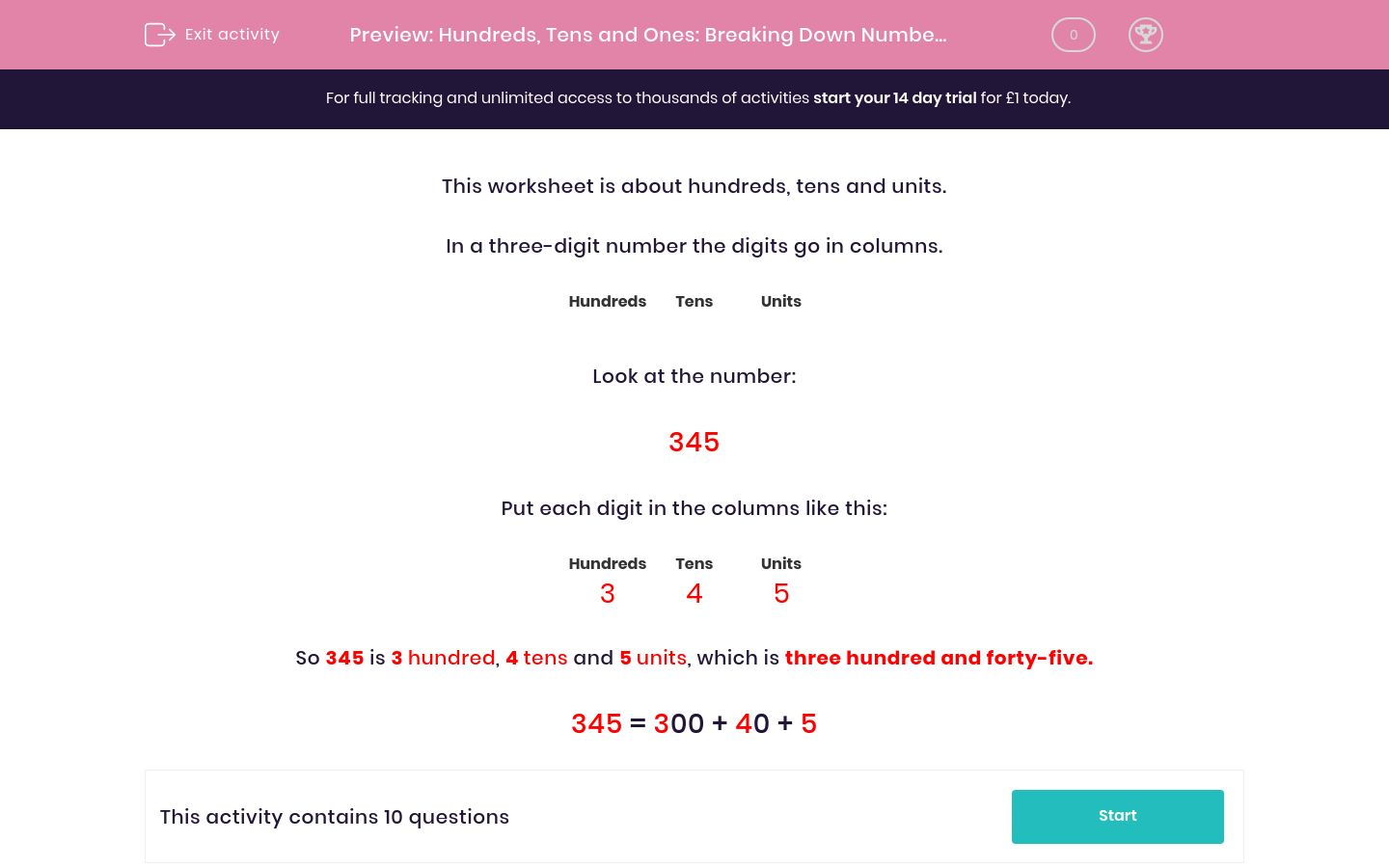# Hundreds, Tens and Ones: Breaking Down Numbers (3)

In this worksheet, students must match numbers to their HTU equivalents.Key stage:  KS 2

Curriculum topic:   Maths and Numerical Reasoning

Curriculum subtopic:   Place Value

Difficulty level:### QUESTION 1 of 10

This worksheet is about hundreds, tens and units.

In a three-digit number the digits go in columns.

 Hundreds Tens Units

Look at the number:

345

Put each digit in the columns like this:

 Hundreds Tens Units 3 4 5

So 345 is 3 hundred, 4 tens and 5 units, which is three hundred and forty-five.

345 = 300 + 40 + 5

Match the numbers to their Hundreds, Tens and Units.

## Column B

539
100 + 30 + 9
239
200 + 30 + 9
139
500 + 30 + 9

Match the numbers to their Hundreds, Tens and Units.

## Column B

139
100 + 30 + 9
199
100 + 80 + 9
189
100 + 90 + 9

Match the numbers to their Hundreds, Tens and Units.

## Column B

479
400 + 70 + 5
476
400 + 70 + 9
475
400 + 70 + 6

Match the numbers to their Hundreds, Tens and Units.

## Column B

839
900 + 80 + 3
398
800 + 30 + 9
983
300 + 90 + 8

Match the numbers to their Hundreds, Tens and Units.

## Column B

839
800 + 80 + 3
898
800 + 30 + 9
883
800 + 90 + 8

Match the numbers to their Hundreds, Tens and Units.

## Column B

839
800 + 30 + 5
836
800 + 30 + 9
835
800 + 30 + 6

Match the numbers to their Hundreds, Tens and Units.

## Column B

537
500 + 30 + 7
375
300 + 70 + 5
753
700 + 50 + 3

Match the numbers to their Hundreds, Tens and Units.

## Column B

232
200 + 30 + 2
292
200 + 80 + 3
283
200 + 90 + 2

Match the numbers to their Hundreds, Tens and Units.

## Column B

557
700 + 50 + 5
377
300 + 70 + 7
755
500 + 50 + 7

Match the numbers to their Hundreds, Tens and Units.

## Column B

245
400 + 20 + 5
254
200 + 40 + 5
542
400 + 50 + 2
524
500 + 40 + 2
452
500 + 20 + 4
425
200 + 50 + 4
• Question 1

Match the numbers to their Hundreds, Tens and Units.

## Column B

539
500 + 30 + 9
239
200 + 30 + 9
139
100 + 30 + 9
EDDIE SAYS
Look at the hundreds.
• Question 2

Match the numbers to their Hundreds, Tens and Units.

## Column B

139
100 + 30 + 9
199
100 + 90 + 9
189
100 + 80 + 9
EDDIE SAYS
Look at the tens.
• Question 3

Match the numbers to their Hundreds, Tens and Units.

## Column B

479
400 + 70 + 9
476
400 + 70 + 6
475
400 + 70 + 5
EDDIE SAYS
Look at the units or ones.
• Question 4

Match the numbers to their Hundreds, Tens and Units.

## Column B

839
800 + 30 + 9
398
300 + 90 + 8
983
900 + 80 + 3
EDDIE SAYS
Look at the hundreds first.
• Question 5

Match the numbers to their Hundreds, Tens and Units.

## Column B

839
800 + 30 + 9
898
800 + 90 + 8
883
800 + 80 + 3
EDDIE SAYS
Look at the tens first.
• Question 6

Match the numbers to their Hundreds, Tens and Units.

## Column B

839
800 + 30 + 9
836
800 + 30 + 6
835
800 + 30 + 5
EDDIE SAYS
Look at the units or ones first.
• Question 7

Match the numbers to their Hundreds, Tens and Units.

## Column B

537
500 + 30 + 7
375
300 + 70 + 5
753
700 + 50 + 3
EDDIE SAYS
Look at the hundreds first.
• Question 8

Match the numbers to their Hundreds, Tens and Units.

## Column B

232
200 + 30 + 2
292
200 + 90 + 2
283
200 + 80 + 3
EDDIE SAYS
Look at the tens first.
• Question 9

Match the numbers to their Hundreds, Tens and Units.

## Column B

557
500 + 50 + 7
377
300 + 70 + 7
755
700 + 50 + 5
EDDIE SAYS
Look at the hundreds first.
• Question 10

Match the numbers to their Hundreds, Tens and Units.

## Column B

245
200 + 40 + 5
254
200 + 50 + 4
542
500 + 40 + 2
524
500 + 20 + 4
452
400 + 50 + 2
425
400 + 20 + 5
EDDIE SAYS
Look at the hundreds first, then the tens, then the units.
---- OR ----

Sign up for a £1 trial so you can track and measure your child's progress on this activity.

### What is EdPlace?

We're your National Curriculum aligned online education content provider helping each child succeed in English, maths and science from year 1 to GCSE. With an EdPlace account you’ll be able to track and measure progress, helping each child achieve their best. We build confidence and attainment by personalising each child’s learning at a level that suits them.

Get started